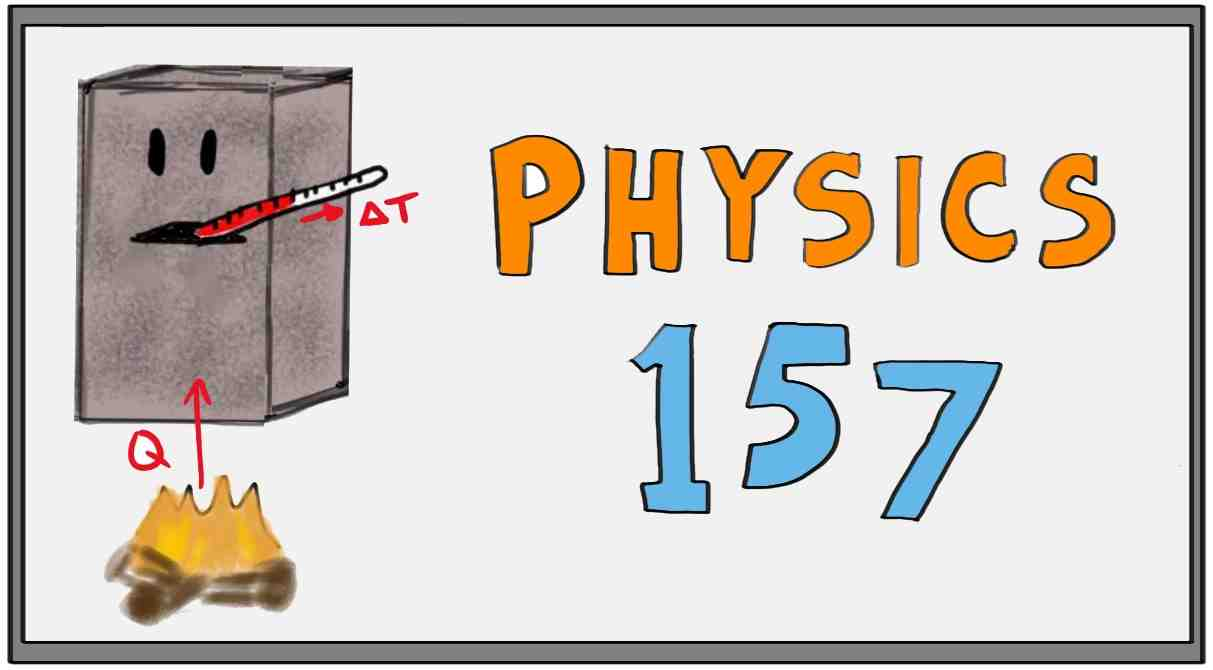Physics 157: Thermodynamics, Oscillations, and Waves

author: Mark Van Raamsdonk (professor of physics at the University of British Columbia)

This page has links to a series of videos on the thermodynamics, oscillations, and waves originally designed for first year engineering students at UBC.

 Topics Video Introduction Lecture 1 video What is thermodynamics? Energy conservation. Effects of heating/cooling on macroscopic properties of objects. IR radiation as a way to "see" microscopic energy. Equilibrium, temperature, and heat. Zeroth Law of Thermodynamics. Lecture 2 video Thermometers and temperature scales. The Celcius scale. Definition of Pressure. Gas thermometers and the ideal gas law. The Kelvin scale. Lecture 3 video Linear relations vs proportionality relations. Kelvin scale. Thermometers. Thermal expansion. Lecture 4 video More thermal expansion, area and volume expansion Lecture 5 video More on volume expansion, Young's modulus, stress, strain Lecture 6 video Thermal stress. Problems with thermal expansion and stress/strain in multipart systems Lecture 7 video Heat capacity, specific  heat. Lecture 8 video Phase diagrams, phase changes,  latent heat. Calorimetry. Lecture 9 video Heat conduction, thermal conductivity. Lecture 10 video More conductivity Lecture 11 video Insulation and R values. Convection and radiation. Electromagnetic waves, spectrum graphs. Lecture 12 video Temperature dependence of spectrum and power emitted for thermal radiation. Emissivity, equilibrium temperature of radiating objects. Lecture 13 video The solar constant, albedo, temperature of the Earth, greenhouse effect. Lecture 14 video Molecular picture of forces from a gas. Ideal gas law. Lecture 15 video First law of thermodynamics. Work Lecture 16 video Work for gases, PV diagrams, Work as area on a PV diagram. Internal energy. Lecture 17 video Internal energy. Free expansion of gas. Calculating heat using the first law. Lecture 18 video Constant volume, constant pressure, constant temperature, and adiabatic processes. Lecture 19 video Review of isochoric, isobaric, isothermal, and adiabatic processes. Adiabatic compression example. Lecture 20 video The internal combustion engine. Example of calculating efficiency. Lecture 21 video The power of an engine. Refrigerators. Lecture 22 video Refrigerators and air conditioners. Why does heat flow from hot to cold? Frogs and entropy. Lecture 23 video Entropy and the Second Law of Thermodynamics. Relation between entropy, heat, and temperature. Lecture 24 video Entropy examples, TS diagrams, calculating efficiency from TS diagrams. Carnot cycle and maximum possible efficiency. Lecture 25 video Mechanical equilibrium, restoring forces, oscillations, simple harmonic motion Lecture 26 video Simple harmonic motion, amplitude, period, angular frequency, velocity, acceleration, phase. Lecture 27 video Displacement/velocity/acceleration in simple harmonic motion Lecture 28 video Simple harmonic motion from small oscillations with non-linear forces. Lecture 29 video Energy in simple harmonic motion, damped oscillations. Lecture 30 video Drag forces, damped oscillations, driven periodic motion, resonance. Lecture 31 video Coupled oscillators, transverse and longitudinal waves, waves on stretched strings, in solids, and in fluids Lecture 32 video Mathematical description of traveling sinusoidal waves. Wavelength, wave number. Relation between wave velocity, period, and wavelength. Lecture 33 video Principle of Superposition, standing waves, wave velocity, constructive and destructive interference Lecture 34 video Applications: musical instruments and gravtational waves Lecture 35 video